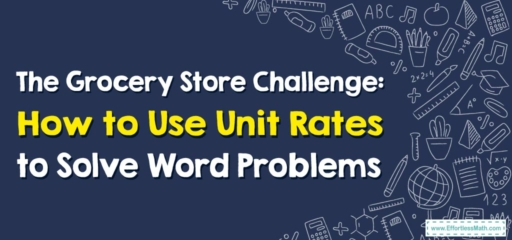# The Grocery Store Challenge: How to Use Unit Rates to Solve Word Problems

Welcome to our real-world math session! Today, we'll navigate the bustling aisles of a grocery store, where unit rates become our best ally to tackle word problems. Strap in for our shopping cart math adventure!## 1. Grocery Shopping with Math: Understanding Unit Rates

In our daily lives, we often make decisions based on unit rates without even realizing it. A unit rate describes how many units of the first type of quantity correspond to one unit of the second type of quantity. For example, if a $$6$$-pack of soda costs $$3$$, the unit rate, or cost per can, is $$\frac{3}{6}$$, which equals $$0.50$$ per can.

## 2. Slaying the Beast: A Guide to Using Unit Rates in Word Problems

### The Shopper’s Guide to Solving Word Problems Using Unit Rates

#### Step 1: Identify the Word Problem

Our mission begins by understanding the problem we’re facing. What are we trying to find? The cost of one item? The speed of a car? The amount of ingredients needed for a recipe?

#### Step 2: Extract the Units and Rates

After identifying the problem, we’ll need to find the given rate. This usually involves two different units, like dollars per soda can, miles per hour, or cups per serving.

#### Step 3: Calculate the Unit Rate

Now, let’s find our unit rate by dividing the first quantity by the second quantity.

#### Step 4: Use the Unit Rate to Solve the Problem

We’ll apply the unit rate to solve our problem. This might mean multiplying the unit rate by a certain number or using the unit rate to compare two different options.

Let’s try an example: You’re in the grocery store and see two brands of orange juice. One brand costs $$3.00$$ for a $$1$$-liter bottle. The other brand is $$5.00$$ for a $$2$$-liter bottle. Which is the better buy?

1. Identify the Word Problem: We’re trying to find which brand of orange juice is cheaper per liter.
2. Extract the Units and Rates: The first brand is $$3.00$$ per liter, and the second brand is $$5.00$$ per $$2$$ liters.
3. Calculate the Unit Rate: The unit rate for the first brand is $$\frac{3.00}{1} = 3.00$$ per liter. The unit rate for the second brand is $$\frac{5.00}{2} = 2.50$$ per liter.
4. Use the Unit Rate to Solve the Problem: The second brand is cheaper per liter, so it’s the better buy!

Congratulations! You’ve successfully navigated the grocery store aisles using unit rates. With this handy tool, you’re well-equipped to make smart, cost-effective decisions. So, happy shopping and happy calculating!

### What people say about "The Grocery Store Challenge: How to Use Unit Rates to Solve Word Problems - Effortless Math: We Help Students Learn to LOVE Mathematics"?

No one replied yet.

X
30% OFF

Limited time only!

Save Over 30%

SAVE $5 It was$16.99 now it is \$11.99